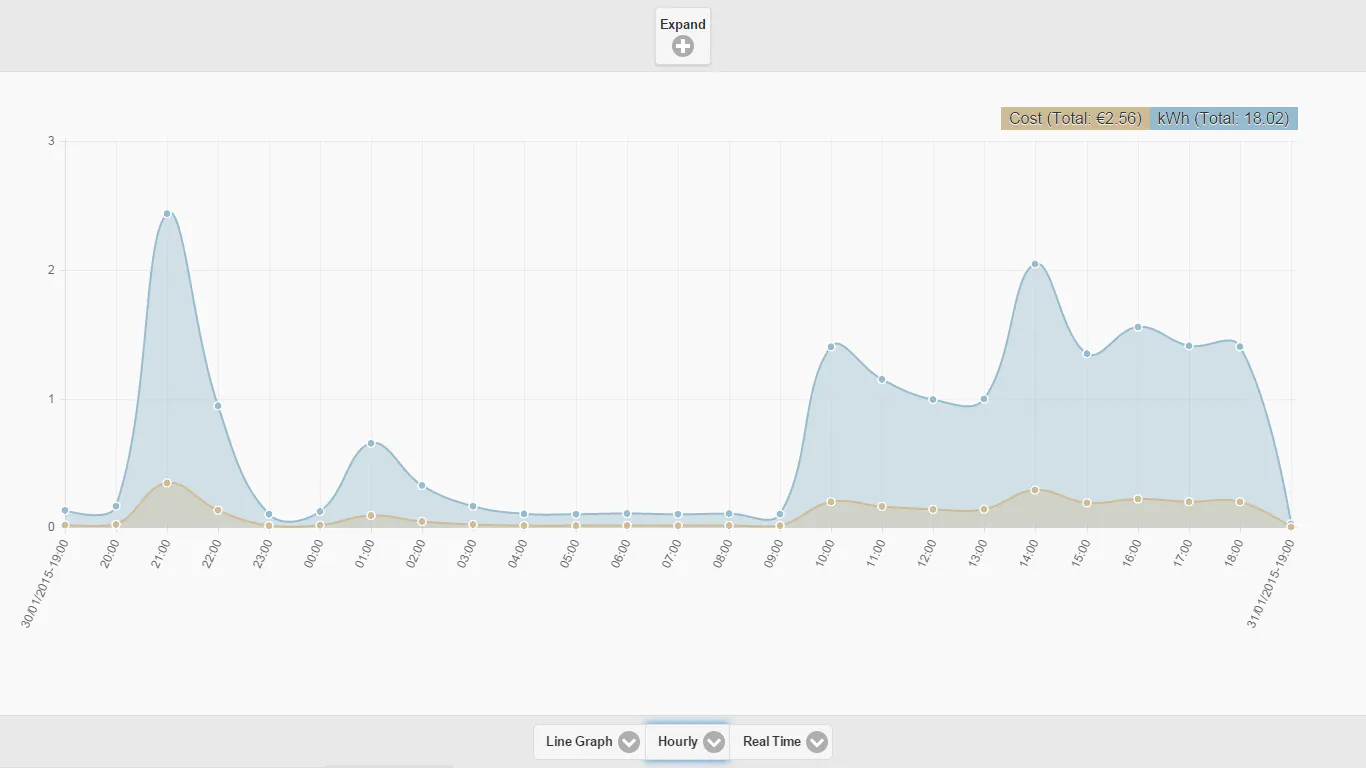Published

# Spark Non-Invasive Smart Electricity Meter

A simple Non-Invasive Smart Electricity Meter using Spark Core

Full instructions provided17,931## Things used in this project

### Hardware components

 Non Invasive current sensor (CT sensor YHDC SCT-013-000 )
×1
 Burden resistor 18 Ohms
×1
 10k Ohm resistors
×2
 10uF capacitor
×1

Read more

## Code

### file_10981.txt

C/C++
The project uses the EmonLib Library for Electricity monitoring  https://github.com/openenergymonitor/EmonLib
```// This #include statement was automatically added by the Spark IDE.

#include "HttpClient/HttpClient.h"
#include "application.h"
#include "EmonLib.h"
#include <string.h>

HttpClient http;
http_request_t request;
http_response_t response;
http_header_t headers[] = {

{ "Content-type", "application/x-www-form-urlencoded" },
{ NULL, NULL } // NOTE: Always terminate headers will NULL
};

EnergyMonitor emon1;                   // Create an instance
TCPClient client;
const int voltage = 220;
double average = 0.0; // interval at which to do something (millisecond)
double sum = 0.0; // interval at which to do something (milliseconds)
int count = 0; // interval at which to do something (milliseconds)

static unsigned long secondInterval = 1000;
static unsigned long minuteInterval = 57000;
static unsigned long prevMinute = 0;
static unsigned long prevSecond = 0;
unsigned long now;

void setup()
{
Serial.begin(9600);
emon1.current(0, 103.1);
}

void loop()

{
unsigned long currentMillis = millis();
if ((unsigned long)(currentMillis - prevSecond) < secondInterval){
return;
}
else{

double Irms = emon1.calcIrms(1480);  // Calculate Irms only
//double watts = (Irms*voltage) - 16;  // Calculate Irms only
double watts = (Irms*1000) ;  // Calculate Irms only
sum = sum + watts;
count = count + 1;
if ((unsigned long)(currentMillis - prevMinute) < minuteInterval){
return;
}
else{
average = sum/count;
sendWatts(average);
average = 0.0;
sum = 0.0;
count = 0;
prevMinute = currentMillis;
}
prevSecond = currentMillis;
}
}

void sendWatts(double wattreading){
String val = "watts=" + doubleToString(wattreading,2);
request.hostname = "yourhost.com";
request.port = 80;
request.path = "/php/insertConsumption.php";
request.body = val ;
http.post(request, response, headers);
}

String doubleToString(double input,int decimalPlaces){
if(decimalPlaces!=0){
String string = String((int)(input*pow(10,decimalPlaces)));
if(abs(input)<1){
if(input>0)
string = "0"+string;
else if(input<0)
string = string.substring(0,1)+"0"+string.substring(1);
}

return string.substring(0,string.length()-decimalPlaces)+"."+string.substring(string.length()-decimalPlaces);

}
else {
return String((int)input);
}

}
```

### file_13723.php

PHP
insertConsumption.php
```<?php

include_once('IConnectInfo.php');

\$link = mysql_connect(IConnectInfo::HOST, IConnectInfo::UNAME, IConnectInfo::PW)

or die('Could not connect: ' . mysql_error());

//echo "Connected successfully \n";

mysql_select_db(IConnectInfo::DBNAME) or die('Could not select database');

if(isset(\$_POST['watts']) ) {

if(ctype_digit(\$_POST['watts']) && is_int(\$_POST['watts'])) {

\$poswatts = \$_POST['watts'];

if(\$poswatts<0 ) \$poswatts  = 0;

date_default_timezone_set("UTC");

\$currutctime = date("Y-m-d H:i:s", time());

\$pdo = new PDO('mysql:store.db');

\$stmt = \$pdo->prepare('INSERT INTO Consumption (watts,unixtime)   VALUES (:watts, :unixtime);');

\$stmt->bindParam(':watts', \$poswatts, PDO::PARAM_INT);

\$stmt->bindParam(':unixtime', \$currutctime, PDO::PARAM_STR);

\$stmt->execute();

} else {

// reject id value and report error to user

}

}

?>

```

### file_12188.php

PHP
insertConsumption.php - (w/o input validation) -
``` <?php

include_once('IConnectInfo.php');

\$link = mysql_connect(IConnectInfo::HOST, IConnectInfo::UNAME, IConnectInfo::PW)

or die('Could not connect: ' . mysql_error());

//echo "Connected successfully \n";

mysql_select_db(IConnectInfo::DBNAME) or die('Could not select database');

// varying values

if(isset(\$_POST['watts']) ) {

\$poswatts = \$_POST['watts'];

if(\$poswatts<0 ) \$poswatts  = 0;

date_default_timezone_set("UTC");

\$currutctime = date("Y-m-d H:i:s", time());

\$sql = 'INSERT INTO Consumption '.

'(watts,unixtime) '.

'VALUES ( '. \$poswatts .', "'. \$currutctime .'");';

\$result = mysql_query(\$sql) or die('Query failed: ' . mysql_error());

echo("success-" .mysql_insert_id());

//mysql_free_result(\$result);

mysql_close(\$link);

//echo(\$result);

}

?>

```

## Credits

### Yonas Leguesse

5 projects • 42 followers